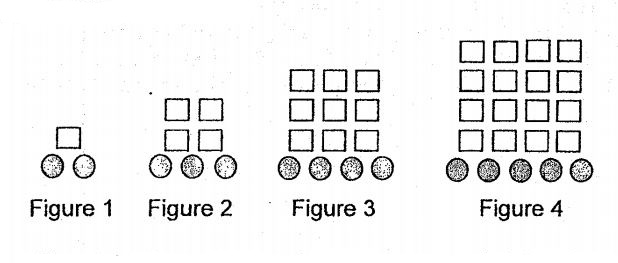# Question 1 of 40

Hatta formed some figures that followed a pattern using squares and circles as shown in figure below.
The table Shows the number of squares and circles for the first four figures. \begin{array}{|c|c|c | c | c |} \hline \mbox{Figure Number} & 1 & 2 & 3 & 4 \\ \hline \mbox{Number of squares} & 1 & 4 & 9 & 16 \\ \hline \mbox{Nunmber of circles} & 2 & 3 & 4 & 5 \\ \hline \mbox{No. of squares divided by No. circles} & 0R1 & 1R1 & 2R1 & 3R1 \\ \hline \end{array} Note: “R” denotes remainder in the above columns.
(a) A Figure has 3481 squares. Find the answer when its number of squares is divided by its number of circles.
(b) In a certain Figure number, 99 R1 is obtained when its number of squares is divided by its number of circles. Find the total number of squares and circles in that Figure number.A
(a) 52R1
(b) 10,000
B
(a) 54R1
(b) 10,010
C
(a) 56R1
(b) 10100
D
(a) 58R1
(b) 10101
E
None of the above